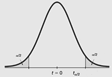# Ch 8.2 Confidence Interval for Mean One Sample No Sigma

$$\newcommand{\vecs}{\overset { \rightharpoonup} {\mathbf{#1}} }$$ $$\newcommand{\vecd}{\overset{-\!-\!\rightharpoonup}{\vphantom{a}\smash {#1}}}$$$$\newcommand{\id}{\mathrm{id}}$$ $$\newcommand{\Span}{\mathrm{span}}$$ $$\newcommand{\kernel}{\mathrm{null}\,}$$ $$\newcommand{\range}{\mathrm{range}\,}$$ $$\newcommand{\RealPart}{\mathrm{Re}}$$ $$\newcommand{\ImaginaryPart}{\mathrm{Im}}$$ $$\newcommand{\Argument}{\mathrm{Arg}}$$ $$\newcommand{\norm}{\| #1 \|}$$ $$\newcommand{\inner}{\langle #1, #2 \rangle}$$ $$\newcommand{\Span}{\mathrm{span}}$$ $$\newcommand{\id}{\mathrm{id}}$$ $$\newcommand{\Span}{\mathrm{span}}$$ $$\newcommand{\kernel}{\mathrm{null}\,}$$ $$\newcommand{\range}{\mathrm{range}\,}$$ $$\newcommand{\RealPart}{\mathrm{Re}}$$ $$\newcommand{\ImaginaryPart}{\mathrm{Im}}$$ $$\newcommand{\Argument}{\mathrm{Arg}}$$ $$\newcommand{\norm}{\| #1 \|}$$ $$\newcommand{\inner}{\langle #1, #2 \rangle}$$ $$\newcommand{\Span}{\mathrm{span}}$$$$\newcommand{\AA}{\unicode[.8,0]{x212B}}$$

### Ch 8.2 Confidence Interval for mean with one sample when sigma is not known.

Use one sample with size n, $$\bar{x}$$, s

$$\fbox { 1) point estimate of \( \mu: \bar{x}$$ } \)

$$\fbox{ 2) Interval estimate of μ: \( \bar{x} - E < \mu < \bar{x} +E$$ } \)

When $$E (EBM) = t_ {\alpha /2} \frac{s}{\sqrt{n}}$$  when σ is not given or unknown.

Use Online calculator to find confidence interval of mean whenis not given.

- use online statdisk: https://www.statdisk.com/#

Analysis/Confidence Intervals/Mean one sample/.

If summary statistics ( $$\bar{x}$$ , s, n) are given, select “use Summary Statistics” tab otherwise use “use data” tab.

Enter Clevel, n, $$\bar{x}$$ , s, or select with data tab.

Do not enter population standard deviation.

Output:  E is the margin of error or Error bound for Mean.

Confidence interval estimate is  $$\bar{x} - E < \mu < \bar{x} +E$$

Note: the requirement for this confidence interval is CTL applies (n > 30 or X is normal). Sample is SRS.

### B) T-distribution

$$t_ {\alpha /2}$$ is the critical value with C-level in the middle.To find  $$t_ {\alpha /2}$$

Use Online Stat book  Inverse-t Calculator: http://onlinestatbook.com/2/calculators/inverse_t_dist.html

the degree of freedom = n – 1,  Select confidence interval from 90%, 95% or 99%. “Calculate”

The “t for confidence interval” is the critical value tα/2.

Properties of t-distributions:

1) $$t = \frac{x-\mu } {s/\sqrt{n}}$$ = no. of times of $$s/\sqrt{n}$$  $$\bar{x}$$  is from mean μ when $$\bar{x}$$ has a normal distribution.

2) Each t-distribution is different for different sample size according to the degree of freedom df = n – 1.

3) Each t-distribution has the same general symmetric bell shape as standard normal, but with more variability.

4) As sample size increase, the student t- distribution approaches the standard normal distribution.

Ex1: Patients with insomnia are treated with zopiclone. After the treatment, the 16 subjects had a mean wake time of 98.9 min. and a sample standard deviation of 42.3 min. Assume that wake times are normally distributed.

a) Construct a 98% confidence interval estimate of mean wake time for all patients treated by zopiclone.

- since X is normal, x-bar is normal, σ is not known, so use t-distribution.

- Identify C-level = 0.98, n= 16, $$\bar{x}$$ = 98.9, s = 42.3,

- Use statdisk/ Analysis/Confidence interval/ Mean one sample/

- output:  E  = 27.521,  Confidence interval is  71.379 < μ <  126.421

interval format (71.379, 126.421),    +/- format: x-bar $$\pm$$ E,  89.9 ± 27.521

b) Can we conclude the mean wake time is less than 100 min.?

No, since the interval is from 71.4 to 126.4, not all values are less than 100 min.

c)  If confidence level is decreased to 95%, what will  happen to the margin of error?

When confidence level decrease,  $$t_{\alpha /2}$$ will decrease, so Margin of error will decrease.

### C) Interpret the Confidence interval of mean:

i) We estimate with  ________ confidence that the true population mean for ______________is between ___ and ___ .

ii) 90% of confidence interval constructed in this way contains the true ______________population mean.

Make conclusion from a confidence Interval:

1) Any value in the confidence Interval can be μ.

2) If the whole interval > a, we can conclude μ > a

3) If the whole interval < a, we can conclude μ < a.

4) When two confidence interval overlap, we can conclude that the two μ may be the same. We cannot conclude one of the μ is higher.

5) Never make conclusion about population mean based solely on value of $$\bar{x}$$ .

Ex2. Listed below are amounts of arsenic (in ug, per serving) in samples of brown rice from California.

5.4, 5.6, 7.3, 4.5, 7.5, 1.5, 5.5, 9.1, 8.7, 8.4

a) Can we assume that amount of arsenic in brown rice is from a normal distribution?

Use Normal Quantile plot to access Normality.

Copy data to statdisk.  Data/Normal AssessmentThe points are reasonably close to a straight line. So we can assume population X is normal.

b) Construct 90% confidence interval of mean arsenic in all brown rice from California. What distribution is sued?

-Since X is normal, so $$\bar{x}$$ is normal, σ is not known, so use t distribution.

-identify Clevel = 0.9, column in statdisk.

- Statdisk Analysis/Confidence intervals/Mean One sample /use data tab.

output:   E  = 1.348 ,  5.002 < mean  < 7.698

-Interval estimate: 5.0 ug < μ < 7.7  ug

c) Find the critical value.

Since t-distribution is used when σ is not known, use online calculator inverse t-calculator.

degree of freedom = 9, C-level = 90% ,   critical value t0.05  = 1.833

d) Interpret the meaning of the confidence Interval:

“We estimate with 90% confidence that the mean amount of arsenic is between 5.0 ug and 7.7 ug.”

“90% of all confidence interval collected from sample of size 10 will contain the true mean arsenic.”

d) Can we conclude the mean amount is less than 8 ug?

Yes, because all values in the interval are less than 8 ug. We can conclude that the mean amount of arsenic in all brown rice are less than 9 ug. at 90% confidence.

Ch 8.2 Confidence Interval for Mean One Sample No Sigma is shared under a not declared license and was authored, remixed, and/or curated by LibreTexts.